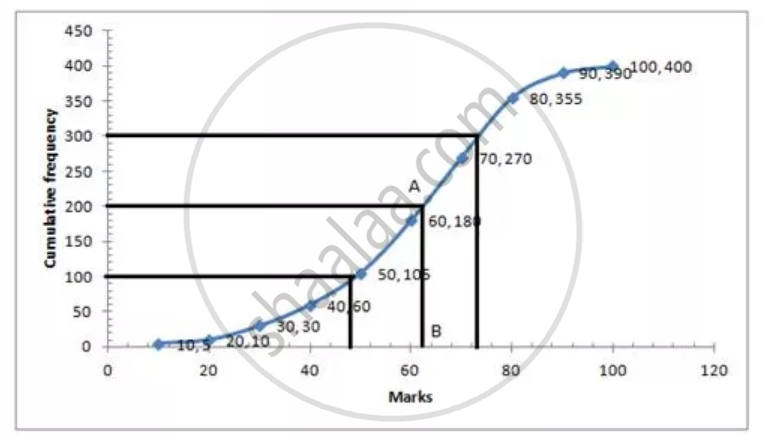Share

# Attempt this Question on a Graph Paper. the Table Shows the Distribution of Marks Gained by a Group of 400 Students in an Examination. - ICSE Class 10 - Mathematics

ConceptMeasures of Central Tendency - Mean, Median, Mode for Raw and Arrayed Data

#### Question

Attempt this question on a graph paper. The table shows the distribution of marks gained by a group of 400 students in an examination.

 Marks (Less than ) 10 20 30 40 50 60 70 80 90 100 No.of student 5 10 30 60 105 180 270 355 390 400

Using scaie of 2cm to represent 10 marks and 2 cm to represent 50 student, plot these point and draw a smooth curve though the point

Estimate from the graph :

(1)the midian marks

(2)the quartile marks

#### Solution

 Marks (less than) No.of students 10 5 20 10 30 30 40 60 50 105 60 180 70 270 80 355 90 390 100 400

Number of terms=400∴ Median= 400/2=200^("th") term

Through  200th term mark draw a line parallel to the x-axis which meets the curve at A. draw a prependiculer to x-axis which meet it at B value of B is the median = 62

Lower Quartile = Q_1=400/4=100^("th") term=49

Upper Quartile= 400xx3/4=300^("th")  term=74

Is there an error in this question or solution?

#### APPEARS IN

Selina Solution for Selina ICSE Concise Mathematics for Class 10 (2018-2019) (2017 to Current)
Chapter 24: Measure of Central Tendency(Mean, Median, Quartiles and Mode)
24C | Q: 11
Solution Attempt this Question on a Graph Paper. the Table Shows the Distribution of Marks Gained by a Group of 400 Students in an Examination. Concept: Measures of Central Tendency - Mean, Median, Mode for Raw and Arrayed Data.
S